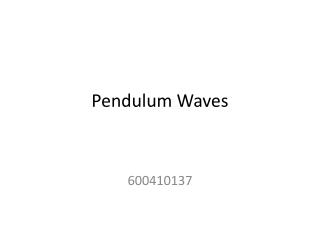# Pendulum Waves - PowerPoint PPT PresentationDownload PresentationPendulum Waves

Pendulum WavesDownload Presentation## Pendulum Waves

- - - - - - - - - - - - - - - - - - - - - - - - - - - E N D - - - - - - - - - - - - - - - - - - - - - - - - - - -
##### Presentation Transcript

1. Pendulum Waves 600410137

2. What is Pendulum Wave

3. What is Pendulum Wave • Many different period pendulums consist of Pendulum Waves. • The length of each pendulum and gravity determine the period of each pendulum. • By varying the length of a series of pendulums, some very interesting patterns emerge.

4. Outline • Method • Environment • Result • Future Work

5. Method • … • :a “Pendulum Wave” period • N:the times of longest pendulum oscillates, the second to longest pendulum oscillates N+1 times • d:The interval distance between each two adjacent pendulums • x:the total distance (from beginning to the end) • We use L(x) to design our length of each pendulum

6. Method • According to L(x) equation and our parameter,we have got the result below.

7. Method • After setting the length of each pendulum and other parameters. We have to simulate how these pendulums wave. • We have to know it’s wave function. • The wave displacement function can be written as

8. Method • The wave displacement function can be written as • :angular frequency, owing to the difference of pendulum length, we can combined it with then we inferred • k: Wave number k at the very beginning of the oscillation. • A:amplitude of the waves • Finally,

9. Environment • Textures make experiment equipment more authentic.(the board the lamp and the bar ) • Also lighting and material parameters make the pendulum looks more heavy , like the real ones. • Yellow: gold • red:ruby • white:pearl • orange:copper (According to OpenGL Material)

10. Environment

11. Environment

12. Result

13. Future Work • We need more accurate calculation, because after the whole pendulum waves loop a few cycles, the displacement of some pendulum strayed out a little bit. • GPU programming maybe suited to this simulation of Pendulum waves, because we can take apart these 12 pendulum waves as parallel process working simultaneously.